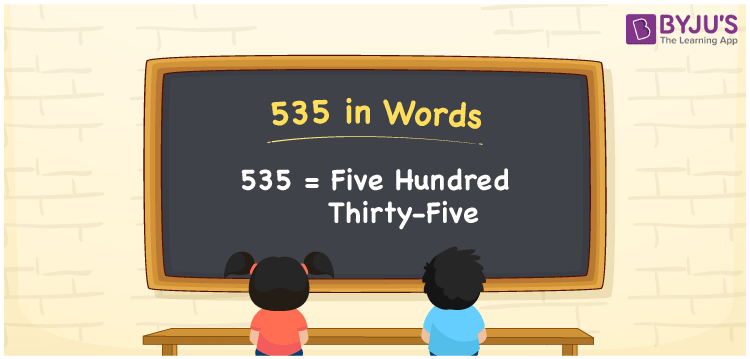# 535 in words

535 in words is written as Five Hundred and Thirty Five. 535 represents the count or value. The article on Counting Numbers can give you an idea about count or counting. The number 535 is a 3 digit number that is used in expressions related to money, days, distance, length, weight and so on. Let us consider an example for 535. “The distance between my school and home is just Five Hundred and Thirty Five meters.”

 535 in words Five Hundred and Thirty Five Five Hundred and Thirty Five in Numbers 535

## 535 in English Words## How to Write 535 in Words?

We can convert 535 to words using a place value chart. The number 535 has 3 digits, so let’s make a chart that shows the place value up to 3 digits.

 Hundreds Tens Ones 5 3 5

Thus, we can write the expanded form as:

5 × Hundred + 3 × Ten + 5 × One

= 5 × 100 + 3 × 10 + 5 × 1

= 535

= Five Hundred and Thirty Five.

535 is the natural number that is succeeded by 534 and preceded by 536.

535 in words – Five Hundred and Thirty Five.

Is 535 an odd number? – Yes.

Is 535 an even number? – No.

Is 535 a perfect square number? – No.

Is 535 a perfect cube number? – No.

Is 535 a prime number? – No.

Is 535 a composite number? – Yes.

## Solved Example

1. Write the number 535 in expanded form

Solution: 5 × 100 + 3 × 10 + 5 × 1

We can write 535 = 500 + 30 + 5

= 5 × 100 + 3 × 10 + 5 × 1.

## Frequently Asked Questions on 535 in words

Q1

### How to write the number 535 in words?

535 in words is written as Five Hundred and Thirty Five.
Q2

### Is 535 divisible by 5?

Yes. 535 is divisible by 5.
Q3

### Is 535 a prime number?

No. 535 is not a prime number.